# HBSE 10th Class Maths Notes Chapter 6 Triangles

Haryana State Board HBSE 10th Class Maths Notes Chapter 6 Triangles Notes.

## Haryana Board 10th Class Maths Notes Chapter 6 Triangles

Introduction
In earlier classes, we have studied the triangles and their properties. We have also studied, the congruence of two geometric figures, and some basic theorems and results on the congruence of triangles. Recall that two figures are said to be congruent, if they have the same shape and same size.

It this chapter, we shall study about those figures which have same shape but not necessarily the same size. Two figures having the same shape but not necessarily of same size are known as similar figures.

Two congruent figures are always similar, but two similar figures may not be congruent.
All regular polygon of same number of sides such as squares, equilateral triangles, rectangles, etc. are similar. All circles are similar but a square and a triangle cannot be similar. In case of two triangles which may appear to be similar but actually, they may not be similar. So, we apply some criteria to determine the similarity of two polygons. In this chapter, we shall study the similarity of triangles.

• A simple closed curve made up of only line segments is called a polygon.
• A polygon of three sides is called a triangle.
• A median of a triangle is a line joining a vertex to the midpoint of the opposite side.
• If corresponding angles of two triangles are equal, then they are known as equiangular triangles.
• The same ratio of the corresponding sides of two polygons is known as the scale factor or the representative fraction for the polygons.
• The angle bisector of a triangle is a line segment that bisects one of the vertex angle of a triangle.
• The altitude of a triangle is a line that extends from one vertex of a triangle and perpendicular to the opposite side.
• The angle of elevation of the Sun is the angle between the direction of the geometric centre of the sun’s apparent disc and the horizontal level.
• A triangle having any three sides of different lengths is called scalene triangle.
• A triangle having any two sides of same lengths is called isosceles triangle.
• A triangle having all three sides of same lengths is called equilateral triangle.
• A triangle having all three angles acute (less than 90°) is called acute angled triangle.
• A triangle having an angle obtuse (greater than 90°) is called obtuse angled triangle.
• A triangle having an angle of measure 90° is called a right angled triangle or right triangle. The perimeter of a triangle is the sum of all its sides.Similar Figures
Two polygons of the same number of sides are similar, if (i) their corresponding angles are equal and (ii) their corresponding sides are in the same ratio (or proportion).
For example : Quadrilateral ABCD and PQRS given below are similar.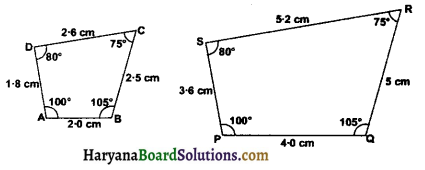We observe that ∠A = ∠P, ∠B = ∠Q, ∠C = ∠R and ∠D = ∠S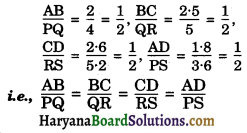Hence, quadrilateral ABCD and PQRS are similar.

Similarity of Triangles
Two triangles are similar, if their
(i) Corresponding angles are equal, and
(ii) Corresponding sides are in the same ratio (or proportion)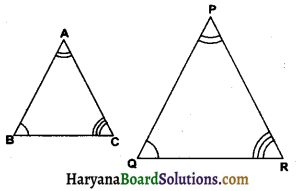For Example: Two triangles ABC and PQR are similar, if
(i) ∠A = ∠P, ∠B = ∠Q, ∠C = ∠R and
(ii) $$\frac{\mathrm{AB}}{\mathrm{PQ}}=\frac{\mathrm{BC}}{\mathrm{QR}}=\frac{\mathrm{AC}}{\mathrm{PR}}$$
If two trianges ABC and PQR are similar we write ΔABC ~ ΔPQR.
A famous Greek mathematician Thales gave an important truth relating to two equiangular triangles which is as follows:
The ratio of any two corresponding sides in two equiangular trianglen is always the same.

Theorem 6.1:
(Basic Proportionality Theorem OR Thales theorem)
If a line is drawn parallel to one side of a triangle to interveet the other two sides in distinct points, the other two sides are divided in the same ratio.
Given: A triangle ABC in which DE || BC and DE intersects AB and AC at D and E respectively.
To Prove: $$\frac{\mathrm{AD}}{\mathrm{DB}}=\frac{\mathrm{AE}}{\mathrm{EC}}$$
Construction : Join BE and CD. Draw EN ⊥ AB and DM ⊥ AC.
Proof : Since EN ⊥ AB. Therefore EN is the height of triangles ADE and DBE.
Now area (ΔADE) = $$\frac{1}{2}$$ base × height
= $$\frac{1}{2}$$ AD × EN
and area (ΔDBE) = $$\frac{1}{2}$$ base × height
= $$\frac{1}{2}$$ BD × EN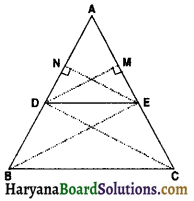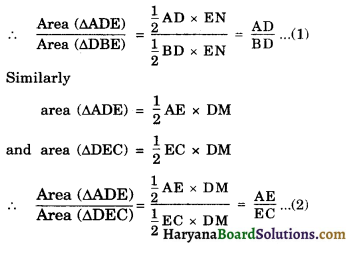But ΔDBE and ΔDEC are on the same base DE and between the same parallels BC and DE
So, Area (ΔDBE) = Area (ΔDEC) …….(3)
Therefore from (1), (2) and (3), we have
$$\frac{\mathrm{AD}}{\mathrm{DB}}=\frac{\mathrm{AE}}{\mathrm{EC}}$$ Proved.Theorem 6.2:
[Converse of Thales Theorem]
If a line divides any two sides of a triangle in the same ratio then the line is parallel to the third side.
Or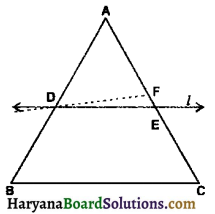Or
In the given figure ABC is atriangle. If $$\frac{\mathrm{AD}}{\mathrm{DB}}=\frac{\mathrm{AE}}{\mathrm{EC}}$$ then prove that DE || BC.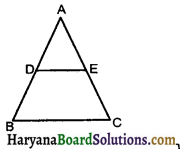Given: A ΔABC and a line l intersecting AB at D and AC at E, such that
$$\frac{\mathrm{AD}}{\mathrm{DB}}=\frac{\mathrm{AE}}{\mathrm{EC}}$$
To Prove: DE || BC
Proof: If possible, Let DE is not parallel te BC, then there must be another line through D which is parallel to BC. Let DF || BC.
∵ DF || BC. Therefore by Basic proportionality theorem, we have,3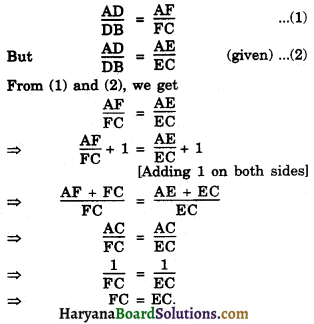This is possible only when E and F coinside.
Hence, DE || BC. Proved

Some Important Results Related to Basic Proportionality Theorem
(a) The internal bisector of an angle of a triangle divides the opposite wide internally in the ratio of the sides containing the angle.
Given: A triangle ABC in which AD is the internal bisector of ∠A
To Prove: $$\frac{\mathrm{BD}}{\mathrm{DC}}=\frac{\mathrm{AB}}{\mathrm{AC}}$$
Construction: Draw CP || DA meets BA produced.
Proof: Since CP || DA and AC is a transversal
∠2 = ∠3 …….. (1)
[alternate Interior angles]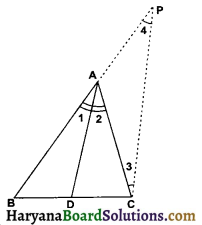CP || DA and BP is a transversal
∠1 = ∠4 …….(2)
[corresponding angles]
But ∠1 = ∠2 …… (3)
From (1), (2) and (3) we get,
∠3 = ∠4
⇒ AP = AC ……(4)
[sides opposite to equal angles are equal]
Now in ΔBCP, DA || CP
⇒ $$\frac{\mathrm{BD}}{\mathrm{DC}}=\frac{\mathrm{AB}}{\mathrm{AP}}$$
[By Basic Proportionality Theorem]
⇒ $$\frac{\mathrm{BD}}{\mathrm{DC}}=\frac{\mathrm{AB}}{\mathrm{AC}}$$ [Using (4)]
Hence $$\frac{\mathrm{BD}}{\mathrm{DC}}=\frac{\mathrm{AB}}{\mathrm{AC}}$$ Proved.(b) The external bisector of an angle of a triangle divides the opposite side externally in the ratio of the sides containing the angle.
Given : A ΔABC such that CE is the bisector of exterior ∠C and intersects AB produced at E.
To Prove: $$\frac{\mathrm{AE}}{\mathrm{BE}}=\frac{\mathrm{CA}}{\mathrm{CB}}$$
Construction : Draw BD || EC Intersecting AC at D.
Proof: Since BD || EC and CB is a transversal
[alternate interior angles]
BD || EC and AC is transversal
∠1 = ∠4 ……(2)
[corresponding angles]
But ∠1 = ∵2 …….(3)
[∵ CE is the bisector of exterior ∠C]
From (1), (2) and (3), we get
∠3 = ∠4 …….(4)
⇒ DC = BC …….(4)
[sides opposite to equal angles are equal]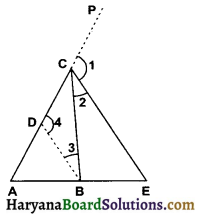In ΔAEC, BD || EC
$$\frac{\mathrm{AB}}{\mathrm{BE}}=\frac{\mathrm{AD}}{\mathrm{DC}}$$
[By Basic Proportionality Theorem]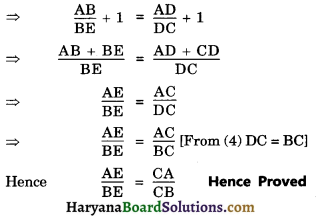Criteria for Similarity of Triangles
We know two triangles are similar, if (i) their corresponding angles are equal and (ii) their corresponding aides are in the same ratio (or proportion)
e.g., In ΔABC and ΔPQR
(i) ∠A = ∠P, ∠B = ∠Q, ∠C = ∠R
(ii) $$\frac{\mathrm{AB}}{\mathrm{PQ}}=\frac{\mathrm{BC}}{\mathrm{QR}}=\frac{\mathrm{CA}}{\mathrm{RP}}$$
Then two triangles are similar.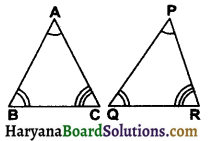Now, we shall discuss some important results which show that if three property choosen out of the six conditions are satisfied, then the other three conditions satisfy automatically, and the two triangles are similar.

Theorem 6.3 (AAA Similarity Criterion)
If in two triangles, the corresponding angles are equal, then their corresponding sides are in the same proportional and hence the two triangles are similar.
Given: Two triangles ABC and DEF such that
∠A = ∠D, ∠B = ∠E And ∠C = ∠F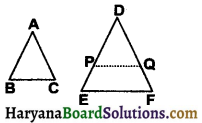To prove : ΔABC ~ ΔDEF
Construction: Cut DP = AB and DQ = AC. Join PQ
Proof: In ΔABC And ΔDPQ, we have
AB = DP (By construction)
AC = DQ (By construction)
∠A = ∠D (given)
ΔABC ≅ ΔDPQ (by SAS congruence)
⇒ ∠B = ∠P (By CPCT)
⇒ ∠E = ∠B [∵ ∠B = ∠E (given)]
But ∠E and ∠P are corresponding angles.
Therefore, PQ || EP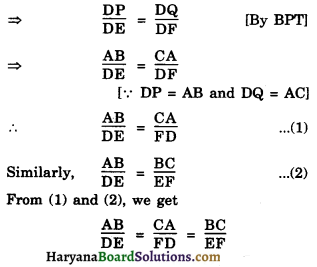and ∠A = ∠D, ∠B = ∠E, ∠C = ∠F (given)
Hence ΔABC ~ ΔDEF Hence Proved
Remark: It follows from the above theorem that two triangles are similar ⇔ They are equiangular.Corollary [AA (angle-angle) similarity criterion]
If two angles of one triangle are respectively equal to two angles of another triangle then the two triangles are similar.
Proof: In triangle ABC and triangle DEF
Let ∠A = ∠D and ∠B = ∠E
We know that sums of 2s of ∠s of triangle is 180°
∴ ∠A + ∠B + ∠C = 180°
and ∠D + ∠E + ∠F = 180°
⇒ ∠A + ∠B + ∠C = ∠D + ∠E + ∠F
⇒ ∠A + ∠B + ∠C = ∠A + ∠B + ∠F
⇒ ∠C = ∠F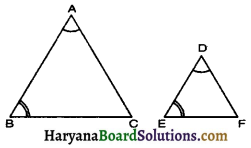Thus, the two triangles are equiangular and hence they are similar.
Remark : AA similarity is the same as AAA similarity.

Theorem 6.4:
[SSS (side-side-side) similarity criterion]
If the corresponding sides of one triangle are proportional to (i.e. in the same ratio) the sides of other triangle, then their corresponding angles are equal and hence the two triangles are similar.
Given: Two triangles ΔABC and ΔDEF such that
$$\frac{\mathrm{AB}}{\mathrm{DE}}=\frac{\mathrm{BC}}{\mathrm{EF}}=\frac{\mathrm{AC}}{\mathrm{DF}}$$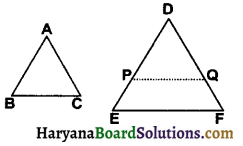To Prove: ΔABC ~ ΔDEF
Construction: Let is take ΔABC and ΔDEF such that
$$\frac{\mathrm{AB}}{\mathrm{DE}}=\frac{\mathrm{BC}}{\mathrm{EF}}=\frac{\mathrm{AC}}{\mathrm{DF}}$$ (<1)
cut DP = AB and DQ = AC. Join PQ
Proof: $$\frac{\mathrm{AB}}{\mathrm{DE}}=\frac{\mathrm{AC}}{\mathrm{DF}}$$
$$\frac{\mathrm{DP}}{\mathrm{DE}}=\frac{\mathrm{DQ}}{\mathrm{DF}}$$
[∵ AB = DP and AC = DQ]
PQ || EF [By converse of BPT]
∠P = ∠E [corresponding angles] …….(1)
and ∠Q = ∠F [corresponding angles] …….(2)
⇒ $$\frac{\mathrm{DP}}{\mathrm{DE}}=\frac{\mathrm{PQ}}{\mathrm{EF}}$$
⇒ $$\frac{\mathrm{AB}}{\mathrm{DE}}=\frac{\mathrm{PQ}}{\mathrm{EF}}$$ ……(3) [∵ DP = AB]
But
⇒ $$\frac{\mathrm{AB}}{\mathrm{DE}}=\frac{\mathrm{BC}}{\mathrm{EF}}$$ ……(4) (given)
From (3) and (4), we get
$$\frac{\mathrm{PQ}}{\mathrm{EF}}=\frac{\mathrm{BC}}{\mathrm{EF}}$$ BC ⇒ BC = PQ
AB = DP and AC = DQ (by construction)
Thus, AB = DP, AC = DQ, BC = PQ
∴ ΔABC ≅ ΔDPQ [By SSS Congruence]
∴ ∠A = ∠D, ∠B = ∠P, ∠C = ∠Q. [By CPCT]
But ∠P = ∠E
and ∠Q = ∠F [From (1) And (2)]
∠A = ∠D, ∠B = ∠E , ∠C = ∠F
Thus, ΔABC ~ ΔDEF
[by AAA Similarity criterion]
Hence Proved

Theorem 6.5:
[SAS (side angle side similarity)]
If one angle of a triangle is equal to one angle of the other triangle and the sides including these angles are proportional, then the two triangles are similar.
Given: Two triangles ΔABC and ΔDEF such that ∠A = ∠D and $$\frac{\mathrm{AB}}{\mathrm{DE}}=\frac{\mathrm{AC}}{\mathrm{DF}}$$
To Prove: ΔABC ~ ΔDEF
Construction: Cut DP = AB and DQ = AC. Join PQ.
Proof: In ΔABC and ΔDPQ, we have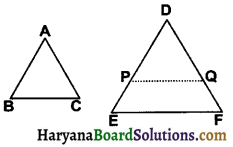AB = DP [by construction]
∠A = ∠D (given)
and AC = DQ [by construction]
∴ ΔABC ≅ ΔDPQ [By SAS congruence]
∠A = ∠D, ∠B = ∠P and ∠C = ∠Q [By CPCT] ……. (1)
Now $$\frac{\mathrm{AB}}{\mathrm{DE}}=\frac{\mathrm{AC}}{\mathrm{DF}}$$ (given)
$$\frac{\mathrm{DP}}{\mathrm{DE}}=\frac{\mathrm{DQ}}{\mathrm{DF}}$$
[∵ AB = DP and AC = DQ]
PQ || EF [By converse of BPT]
∠P = ∠E [Corresponding ∠s] …(2)
and ∠Q = ∠F
From (1) and (2), we get
∠A = ∠D, ∠B = ∠E and ∠C = ∠F
Thus ΔABC ~ ΔDEF.
[by AAA Similarity criterion]
Hence Proved
Remark: If two triangles ΔABC and ΔDEF are similar, then
$$\frac{\mathrm{AB}}{\mathrm{DE}}=\frac{\mathrm{BC}}{\mathrm{EF}}=\frac{\mathrm{AC}}{\mathrm{DF}}$$
⇒ $$\frac{\mathrm{AB}}{\mathrm{DE}}=\frac{\mathrm{BC}}{\mathrm{EF}}=\frac{\mathrm{AC}}{\mathrm{DF}}=\frac{\mathrm{AB}+\mathrm{BC}+\mathrm{AC}}{\mathrm{DE}+\mathrm{EF}+\mathrm{DF}}$$
[By ratio and proportion]
$$\frac{\mathrm{AB}}{\mathrm{DE}}=\frac{\mathrm{BC}}{\mathrm{EF}}=\frac{\mathrm{AC}}{\mathrm{DF}}=\frac{\text { Perimeter of } \triangle \mathrm{ABC}}{\text { Perimeter of } \triangle \mathrm{DEF}}$$Area of Similar Triangles
In this section, we shall discuss the relationship between the ratio of the areas of two similar triangles and the ratio of their corresponding sides. This is stated as follows.

Theorem 6.6:
The ratio of the areas of two similar triangles is equal to the square of the ratio of their corresponding sides.
Given: Two triangles ABC and DEF such that ΔABC ~ ΔDEF
To Prove :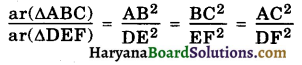Construction : Draw AL ⊥ BC and DM ⊥ EF.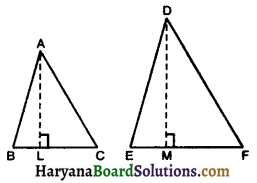Proof : Since ΔABC ~ ΔDEF, it follows that they are equiangular and their corresponding sides are proportional.
∠A = ∠D, ∠B = ∠E, ∠C = ∠F.
and $$\frac{\mathrm{AB}}{\mathrm{DE}}=\frac{\mathrm{BC}}{\mathrm{EF}}=\frac{\mathrm{AC}}{\mathrm{DF}}$$ …..(1)
Thus in ΔALC and ΔDMF.
∠ALC = ∠DMY (each = 90°)
∠C = ∠F (given)
∴ ΔALC ~ ΔDMF (By AA similarity criterion)
⇒ $$\frac{A L}{D M}=\frac{A C}{D F}$$
[Corresponding sides of two similar Δs proportional] …..(2)
From (1) and (2), we get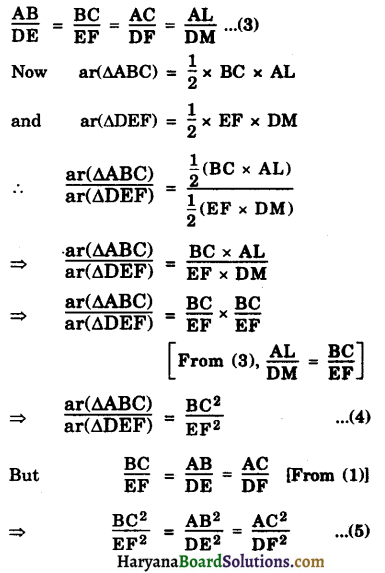Therefore, from (4) and (5), we get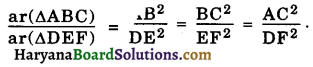Hence Proved

Remark: According to theorem 6.6. we shall prove that areas of two similar triangles are in the ratio of the squares of the corresponding
(i) Altitudes (ii) Medians (iii) Angle bisectors.
(i) Given : ΔABC ~ ΔDEF
AM ⊥ BC and DN ⊥ EF.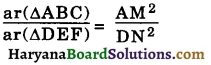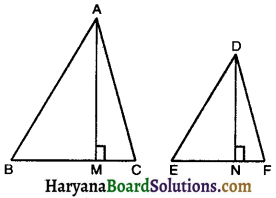Proof: In ΔAMC and ΔDNP
∠AMC = ∠DNF [each = 90°]
∠C = ∠F [∵ ΔABC ~ ΔDEF]
ΔAMC ~ ΔDNF [By AA similarity criterion]
$$\frac{\mathrm{AC}}{\mathrm{DF}}=\frac{\mathrm{AM}}{\mathrm{DN}}$$
[Corresponding sides of similar Δs are proportional] …..(1)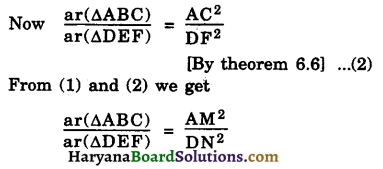Hence Proved

(ii) Given: AX and DY are the median a to side BC and EF respectively.
To Prove :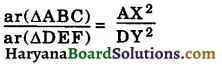Proof : ΔABC ~ ΔDEF (given)
∴ $$\frac{\mathrm{AB}}{\mathrm{DE}}=\frac{\mathrm{BC}}{\mathrm{EF}}=\frac{\mathrm{AC}}{\mathrm{DF}}$$
(corresponding sides of similar Δs are proportional)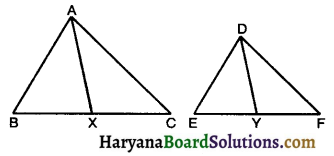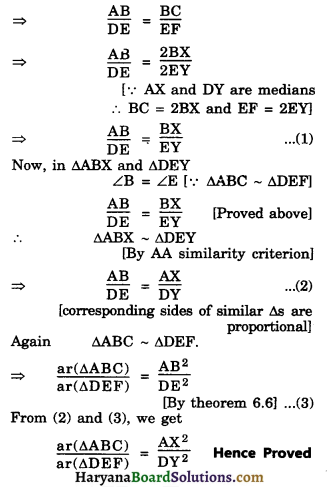(iii) Given ΔABC ~ ΔDEF and AM is the bisector of ∠A and DN is the bisector of ∠D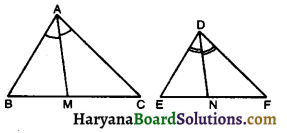To Prove: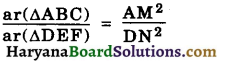Proof: ∵ ΔABC ~ ΔDEF
∴ ∠A = ∠D
[Corresponding ∠s of similar Δs are equal]
⇒ $$\frac{1}{2}$$∠A = $$\frac{1}{2}$$∠D
⇒ ∠CAM = ∠FDN
[∵ AM is the bisector of ∠A and DN is the bisector of ∠D]
Now, in ΔAMC and ΔDNF
∠C = ∠F [∵ ΔABC ~ ΔDEF]
and ∠CAM = ∠FDN [Proved above]
∴ ΔAMC ~ ΔDNF
[By AA similarity criterion]
$$\frac{\mathrm{AC}}{\mathrm{DF}}=\frac{\mathrm{AM}}{\mathrm{DN}}$$ …..(1)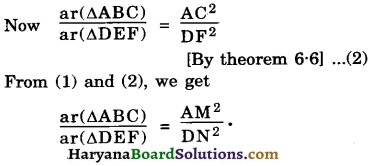Hence ProvedPythagoras Theorem
We have study about Pythagoras theorem in previous classes. We shall prove this theorem using the concept of similarity of triangles. We shall use an important result related to similarity of two triangles formed by the perpendicular to the hypotenuse drawn from the opposite vertex to the hypotenuse of a right triangle. This is stated as follows:

Theorem 6.7:
If perpendicular is drawn from the vertex of the right angle of a right triangle to the hypotenune then triangles on both sides of the perpendicular are similar to the whole triangle and to each other.
Given: Aright ΔABC right angled at ∠B and BD ⊥ AC.
To Prove: (i) ΔADB ~ ΔABC (ii) ΔBDC ~ ΔABC (III) ΔADB ~ ΔBDC
Proof: (i) In ΔADB and ΔABC,
∠ADB = ∠ABC (∵ ∠B = 90° and BD ⊥ AC)
∠A = ∠A (common)
[By AA similarity criterian] … (1)
Hence Proved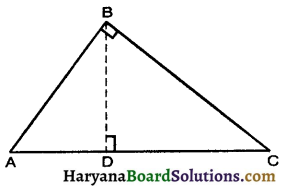(ii) In ΔBDC and ΔABC
∠BDC = ∠ABC (each is 90°)
∠C = ∠C (common)
ΔBDC ~ ΔABC ……(2) [By AA similarity criterion]
Hence Proved

(iii) From (1) and (2), we get
A very important property of a right triangle was given by a Greek mathematician Phythagoras more than 2000 years ago, which is called the Pythagoras theorem. Thus is stated as follows:

Theorem 6.8:
In a right triangle the square of the hypotenuse is equal to the sum of the squares of the other two sides.
Given: A right triangle ΔABC right angled at B.
To Prove (Hypotenuse)2 = (Base)2 + (Perpendicular)2
i.e., AC2 = AB2 + BC2
Construction : Draw BD ⊥ AC.
Proof: ΔADB ~ ΔABC [By theorem 6.7]
⇒ $$\frac{\mathrm{AD}}{\mathrm{AB}}=\frac{\mathrm{AB}}{\mathrm{AC}}$$
[Corresponding sides of similar Δs are proportional]
⇒ AD × AC = AB2 …….(1)
and ΔBDC ~ ΔABC [By theorem 6.7]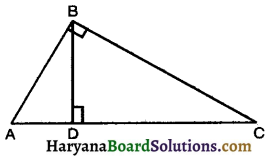⇒ $$\frac{C D}{B C}=\frac{B C}{A C}$$
Corresponding sides of similar Δs are proportional]
⇒ CD × AC = BC2 …..(2)
Adding equations (1) and (2), we get
AD × AC + CD × AC = AB2 + BC2
⇒ AC(AD + CD) = AB2 + BC2
⇒ AC × AC = AB2 + BC2
⇒ AC2 = AB2 + BC2
Hence Proved
This theorem was given by an ancient Indian mathematician Baudhayan about (800 BC) in the following form:
The diagonal of a rectangle produces by itself the same area as produced by its both sides (i.e., length and breadth)
For this reason this theorem is sometimes also referred to as Baudhayan theorem.

Theorem 6.9:
[Converse of Pythagoras theorem]
In a triangle, if square of one side is equal to the sum of the squares of the other two sides, then the angle opposite the first side is a right angle.
Given: A ΔABC such that AC2 + AB2 + BC2
To Prove: ∠B = 90°
Construction : Construct a ΔDEF such that DE = AB, EF = BC and ∠E = 90°
Proof: In ΔDEP, ∠E = 90°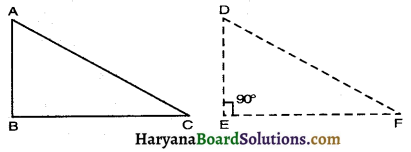So, by the Pythagoras theorem, we have
DF2 = DE2 + EF2
DF2 = AB2 + BC2 ……(1)
[By construction, DE = AB, EF = BC]
But, AC2 = AB2 + BC2 ……(2) (given)
From (1) and (2) we get
DF2 = AC2
⇒ DF = AC ……(3)
Thus, in ΔABC and ΔDEF
AB = DE [By construction]
BC = EF [By construction]
AC = DF [From (3)]
ΔABC ≅ ΔDEF
[By SSS congruente criterian]
⇒ ∠B = ∠E [By CPCT]
⇒ ∠B = ∠E = 90° [∵ ∠E = 90°]
Hence Proved

Some Results related to Pythagoras Theorem
(1) If ΔABC is an abtuse Δ, obtuse angled at B and AD ⊥ CB (Produced), Prove that AC2 = AB2 + BC2 + 2BC × BD.
Given: An obtuse ΔABC in which ∠ABC > 90° and AD ⊥ CB (Produced)
To Prove: AC2 = AB2 + EC2 + 2BC × BD
∴ AB2 = AD2 + BD2 …….(i)
[By Pythagoras theorem]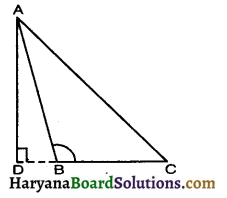∴ AC2 = AD2 + CD2
[By Pythagoras theorem]
⇒ AC2 = AD2 + (BC + BD)2
⇒ AC2 = AD2 + BC2 + BD2 + 2BC ×BD
⇒ AC2 = (AD2 + BD2) + BC2 + 2BC × BD
⇒ AC2 = AB2 + BC2 + 2BC × BD [Using (i)]
Hence Proved

(2) In a ΔABC, ∠B is an acute angle and AD ⊥ BC, Prove that
AC2 = AB2 + BC2 – 2BC × BD.
Given: A ΔABC in which ∠B < 90° and AD ⊥ BC
To Prove: AC2 = AB2 + BC2 – 2BC × BD
∴ AB2 = AD2 + BD2
[By Pythagoras theorem]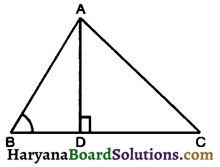∴ AC2 = AD2 + CD2 …..(ii)
[By Pythagoras theorem]
⇒ AC2 = AD2 + [BC – BD]2
⇒ AC2 = AD2 + BC2 + BD2 – 2BC × BD
⇒ AC2 = (AD2 + BD2) + BC2 – 2BC× BD
⇒ AC2 = AB2 + BC2 – 2BC × BD [Using (1)]
Hence Proved

(3) Prove that in any triangle them of the squares of any two sides is equal to twice the square of half of the third side together with twice the square of the median which bisects the third side.
Glven: A triangle ABC in which AD is median
To Prove : AB2 + AC2 = 2AD2 + 2($$\frac{1}{2}$$BC)2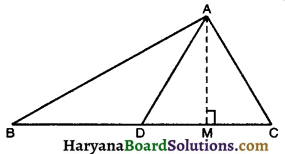Or AB2 + AC2 = 2AD2 + 2BD2
⇒ AB2 + AC2 = 2(AD2 + BD2)
Construction : Draw AM ⊥ BC
Proof: In ΔAMD, ∠AMD – 90°
∴ ∠ADB > 90° and AM ⊥ BD produced
∴ AB2 = AD2 + BD2 + 2BD × DM ……(i) [By Result (1)]
∴ AC2 = AD2 + CD2 – 2CD × DM [By Result (2)]
AC2 = AD2 + BD2 – 2BD × DM ……(ii) [CD = BD]
Addding (i) and (ii), we get
AB2 + AC2 = AD2 + BD2 + 2BD × DM + AD2 + BD2 – 2BD × DM.
⇒ AB2 + AC2 = 2AD2 + 2BD2,
OR, AB2 + AC2 = 2AD2 + 2($$\frac{1}{2}$$BC)2
[∵ BD = $$\frac{1}{2}$$BC]
Hence Proved(4) Prove that three times the sum of the squares of the sides of a triangle is equal to four times the sum of the squares of the medians of the triangle.
Given: A triangle ABC in which AD, BE and CF are three medi
To Prove: 3(AB2+ BC2 + CA2) = 4(AD2 + BE2 + CF2)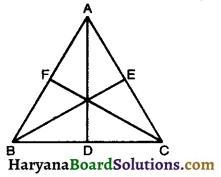Proof: In ΔABC, AD is the median
AB2 + AC2 = 2(AD2 + BD2) [By Result (3)]
⇒ AB2 + AC2 = 2AD2 + $$\frac{1}{2}$$BC2
⇒ AB2 + AC2 = 2AD2 + $$\frac{1}{2}$$BC
⇒ 2AB2 + 2AC2 = 4AD2 + BC2 ….(i)
Similarly in ΔABC, BE and CF are the mediana, we get
2AB2 + 2BC2 = 4BE2 + AC2 ……(ii)
and 2AC2 + 2BC2 = 4CF2 + AB2 ……(iii)
Adding (i), (ii) and (iii), we get
4AB2 + 4BC2 + 4AC2 = 4AD2 + 4BE2 + 4CF2 + BC2 + AC2 + AB2
3AB2 + 3BC2 + 3AC2 = 4AD2 + 4CF2
3(AB2 + BC2 + AC2) = 4(AD2 + BE2 + CF2)
Hence Proved.